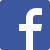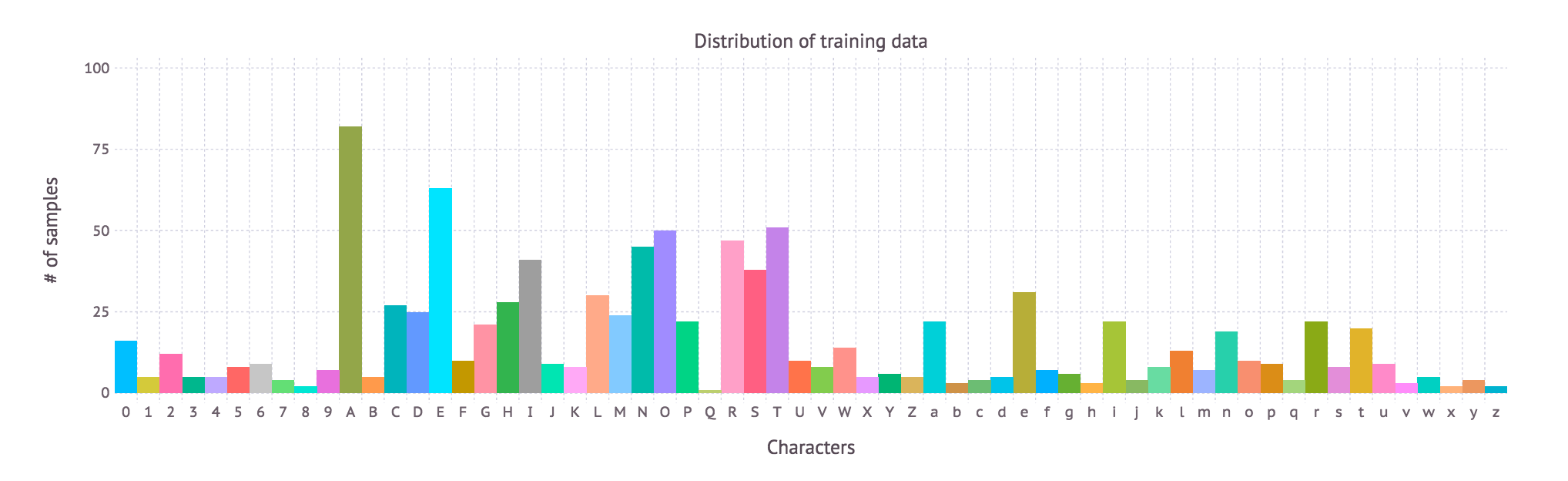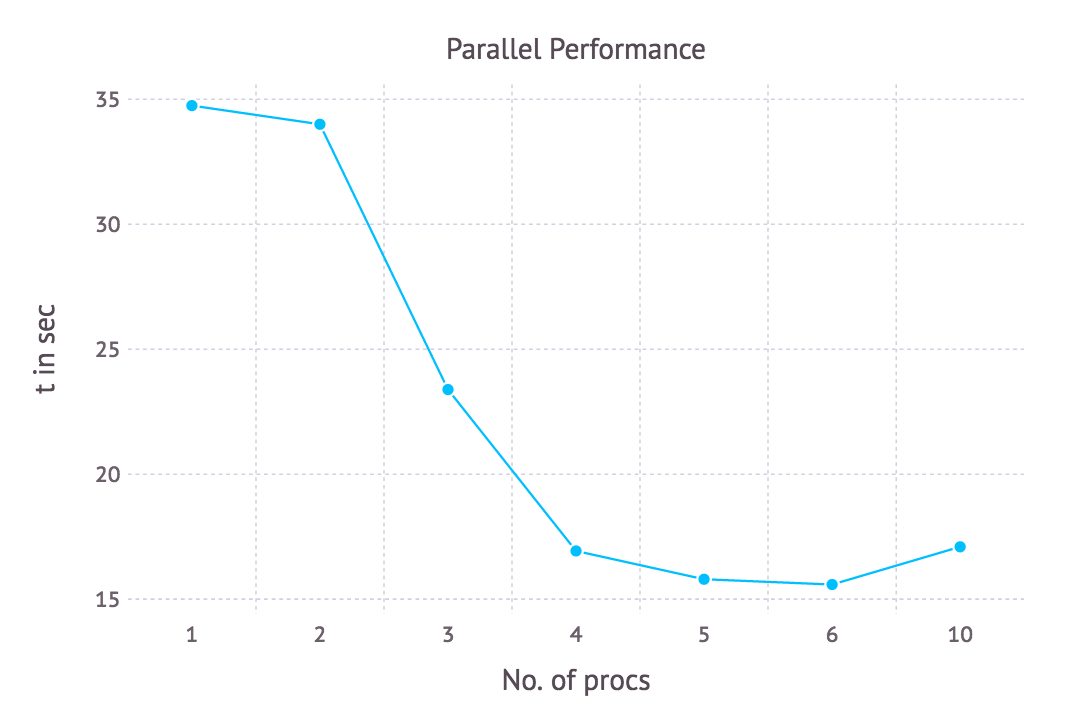Here is the latest from Julia Computing
BLG# An Introduction to Machine Learning in Julia

28 Sep 2016 | Abhijith Chandraprabhu and Ranjan Anantharaman

Machine learning is now pervasive in every field of inquiry and has lead to breakthroughs in various fields from medical diagnoses to online advertising. Practical machine learning is quite computationally intensive, whether it involves millions of repetitions of simple mathematical methods such as Euclidian Distance or more intricate optimizers or backpropagation algorithms. Such computationally intensive techniques need a fast and expressive language – one that enables scientists to write simple, readable code that performs well. Enter Julia.

In this post, we introduce a simple machine learning algorithm called K Nearest Neighbors, and demonstrate certain Julia features that allow for its easy and efficient implementation. We will demonstrate that the code we write is inherently generic, and show the use of the same code to run on GPUs via the ArrayFire package.

## Problem statement

We need to come up with a character recognition software that can identify images of textual characters. The data we’ll be using for this problem are a set of images from Google Street View. The algorithm we’ll be using is called k-Nearest Neighbors (kNN), which is a useful starting point for working on problems of this nature.

## Solution

Let us begin by taking a good look at our data. First, we read the data from a set of CSV files from disk, and plot a histogram that represents the distribution of the training data:

# Read training set
trainLabels = readtable("$(path)/trainLabels.csv") counts = by(trainLabels, :Class, nrow); plot(x = counts[:Class], y = counts[:x1], color = counts[:Class], Theme(key_position = :none), Guide.xlabel("Characters")When developing this code within an integrated environment such as IJulia Notebooks, we can explore this data interactively. For example, we can scroll through the images using the Interact package: @manipulate for i = 1:size(trainLabels,1) load("$(path)/trainResized/\$i.Bmp")
end


### kNN

The solution of labelling the image with its textual character involves finding the “distances” of each image in the training set to every other image. Then, the image under consideration is classified as having the most common label among its k nearest images. We use Euclidean distance to measure the proximity of the images:

Let X be a set of m training samples, Y be the labels for each of those samples, and x be the unknown sample to be classified. We perform the following steps:

• First, calculate all the distances:
for i = 1 to m
distance = d(X(i), x)
end


We can also represent this generally in matrix notation:

function get_all_distances(imageI::AbstractArray, x::AbstractArray)
diff = imageI .- x
distances = vec(sum(diff .* diff,1))
end

• Then sort the distances in ascending order and select first k indices from the sorted list.
function get_k_nearest_neighbors(x::AbstractArray, imageI::AbstractArray, k::Int = 3, train = true)
nRows, nCols = size(x)
distances = get_all_distances(imageI, x)
sortedNeighbors = sortperm(distances)
if train
return kNearestNeighbors = Array(sortedNeighbors[2:k+1])
else
return kNearestNeighbors = Array(sortedNeighbors[1:k])
end
end

• Finally, classify x as the most common element among the k indices.
function assign_label(x::AbstractArray, y::AbstractArray{Int64}, imageI::AbstractArray, k, train::Bool)
kNearestNeighbors = get_k_nearest_neighbors(x, imageI, k, train)
counts = Dict{Int, Int}()
highestCount = 0
mostPopularLabel = 0
for n in kNearestNeighbors
labelOfN = y[n]
counts[labelOfN] = 0
end
counts[labelOfN] += 1
if counts[labelOfN] > highestCount
highestCount = counts[labelOfN]
mostPopularLabel = labelOfN
end
end
mostPopularLabel
end


The objective is to find the best value of k such that we reach optimal accuracy.

### Training

We use the labeled data to find the optimal value of k. The following code will output the accuracy for values of k from 3 to 5, for example.

for k  = 3:5
checks = trues(size(train_data, 2))
@time for i = 1:size(train_data, 2)
imageI = train_data[:,i]
checks[i]  = (assign_label(train_data, trainLabelsInt, imageI, k, true) == trainLabelsInt[i])
end
accuracy = length(find(checks)) / length(checks)
@show accuracy
end


### Testing

Now, from our training, we should have identified a value of k that gives us the best accuracy. Of course, this varies depending on the data. Suppose our runs tell us the best value of k is 3. It’s time to now test our model. For this, we can actually use the same code that we used for training, but we needn’t check for accuracy or loop over values of k.

x = test_data
xT = train_data
yT = trainLabelsInt
k = 3, #say
prediction = zeros(Int,size(x,2))
@time for i = 1:size(x,2)
imageI = x[:,i]
prediction[i]  = assign_label(xT, yT, imageI, k, false)
end


# Improving Performance

There are a couple of ways we can speed up our code. Notice that each iteration of the for-loop in the main compute loop is independent. This looks like a great candidate for parallelisation. So, we can spawn a couple of Julia processes and execute the for loop in parallel.

## Parallel for-loops

The macro @parallel will split the iteration space amongst the available Julia processes and then perform the computation in each process simultaneously.

Let us first add n processes by using the addprocs function. We then execute the following for loop in parallel.

addprocs(n)
@time sumValues = @parallel (+) for i in 1:size(xTrain, 2)
assign_label(xTrain, yTrain, k, i) == yTrain[i, 1]
end


The following scaling plot shows performance variation as you spawn more processes:## GPU Acceleration

The ArrayFire package enables us to run computations on various general purpose GPUs from within Julia programs. The AFArray constructor from this package converts Julia vectors to ArrayFire Arrays, which can be transferred to the GPU. Converting Arrays to AFArrays allows us to trivially use the same code as above to run our computation on the GPU.

checks = trues(AFArray{Bool}, size(train_data, 2))
train_data = AFArray(train_data)
trainLabelsInt = AFArray(trainLabelsInt)
for k  = 3:5
@time for i = 1:size(train_data, 2)
imageI = train_data[:,i]
checks[i]  = (assign_label(train_data, trainLabelsInt, imageI, k, true) == trainLabelsInt[i])
end
accuracy = length(find(checks)) / length(checks)
@show accuracy
end


Benchmarking against the serial version shows a significant speedup, which has been achieved without much extra code!

Serial Time ArrayFire Time
34 sec 20 sec

# Conclusion

• Julia allows for easy prototyping and deployment of machine learning models.
• Parallelization also very easy to implement.
• Built-in parallelism gives the programmer a variety of options to speed up their code.
• Generic code can be run on GPUs using the package ArrayFire

# Future Work

You now know how to write a simple image recognition model using kNN. While this represents a good starting point for problems such as these, it is by no means the state of the art. We shall explore more sophisticated methods, such as deep neural networks, in a future blog post.

## Recent posts

CSV Reader Benchmarks: Julia Reads CSVs 10-20x Faster than Python and R
22 Jun 2020 | Deepak Suresh
JuliaTeam v2.0 Now Available
12 Jun 2020 | Julia Computing